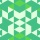Home
IT Knowledge
Inspiration
Languages
EN

# Python - math.tan() method example

0 points
Created by:eyllanesc
277

`math.tan()` is a method that takes only one parameter and returns the approximated value of the tangent mathematical function.

``````import math

print( math.tan( 0                  ) ) #    0 <-    0 degrees

print( math.tan( 0.7853981633974483 ) ) #   ~1 <-  ~45 degrees ==  pi / 4
print( math.tan( 1.5707963267948966 ) ) #~+inf <-  ~90 degrees ==  pi / 2

print( math.tan(-0.7853981633974483 ) ) #  ~-1 <- ~-45 degrees == -pi / 4
print( math.tan(-1.5707963267948966 ) ) #~-inf <- ~-90 degrees == -pi / 2``````

Note: `0.9999999999999999`, `16331239353195370`, `-0.9999999999999999` and `-16331239353195370` should be equal to `1``+Inf`, `-1` and `-Inf` but they are not because of compuptation precision error.

## 1. Documentation

 Syntax ``math.tan(x)`` Parameters `x` - value in radians. Result value calculated as `tan(x)` mathematical function. Description `math.tan()` is a method that takes only one argument and returns the approximated value of the tangent mathematical function.

``````import math

x1 = 0.0            # beginning of calculation in radians
x2 = math.pi / 2    # ending of calculation radians

dx = math.pi / 9    # calculation step in degrees

Output:

``````tan( 0.0  rad ) =  0.0
tan( 0.3490658503988659  rad ) =  0.36397023426620234
tan( 0.6981317007977318  rad ) =  0.8390996311772799
tan( 1.0471975511965976  rad ) =  1.7320508075688767
tan( 1.3962634015954636  rad ) =  5.671281819617707``````

## 3. Working with degrees

``````import math

def calculate_tan(deg):
radians = (math.pi / 180) * deg

# Example:
x1 = 0.0   # beginning of calculation in degrees
x2 = 90.0  # ending of calculation degrees

dx = 30.0  # calculation step in degrees

deg = x1
while deg <= x2:
y = calculate_tan(deg)
print("tan(", deg, " deg ) = ", y)
deg += dx``````

Output:

``````tan(0 deg) = 0
tan(30 deg) = 0.5773502691896257
tan(60 deg) = 1.7320508075688767
tan(90 deg) = 16331239353195370``````

## 4. Reversed console plot example

``````import math

def print_line(y1, y2, dy, character):
line = ""

y = y1
while y < y2:
line += " "
y += dy

print(line, character)

x1 = -3.14   # begining of sine chart
x2 = 3.14    # end of sine chart

y1 = -4.0
y2 = 4.0

x_steps = 60
y_steps = 60

dx = (x2 - x1) / x_steps  # x axis step
dy = (y2 - y1) / y_steps  # y axis step

if y <= y1 or y >= y2:
print(" ")
else:
print_line(y1, y, dy, 'x')

Output:

``````                                x
x
x
x
x
x
x
x
x
x
x
x
x

x
x
x
x
x
x
x
x
x
x
x
x
x
x
x
x
x
x
x
x
x
x
x
x
x

x
x
x
x
x
x
x
x
x
x
x
x``````

## References

1. Trigonometric functions - Wikipedia